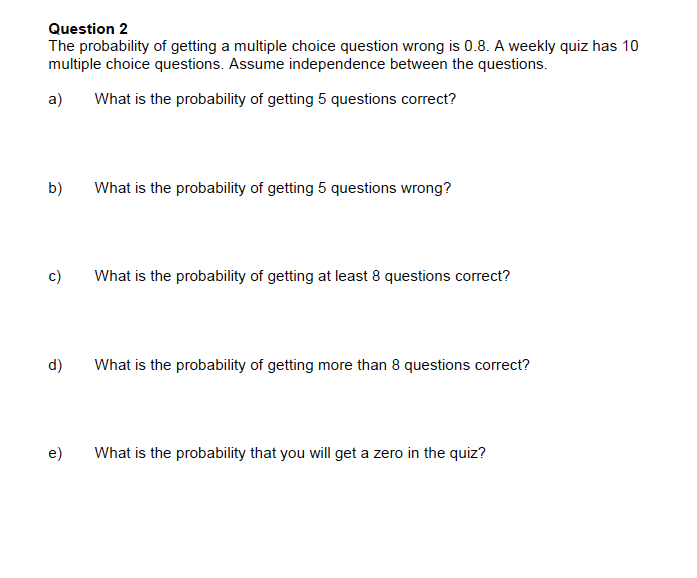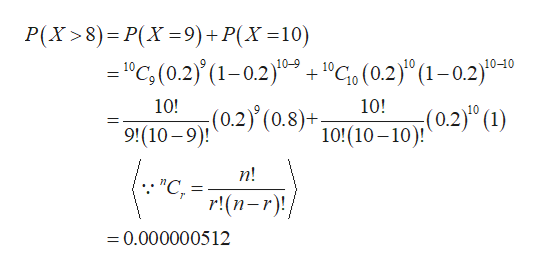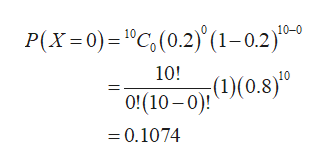Question 2The probability of getting a multiple choice question wrong is 0.8. A weekly quiz has 10multiple choice questions. Assume independence between the questionsa)What is the probability of getting 5 questions correct?b)What is the probability of getting 5 questions wrong?c)What is the probability of getting at least 8 questions correct?d)What is the probability of getting more than 8 questions correct?e)What is the probability that you will get a zero in the quiz?

Question

Need help with part D and E for this question.help_outlineImage TranscriptioncloseQuestion 2 The probability of getting a multiple choice question wrong is 0.8. A weekly quiz has 10 multiple choice questions. Assume independence between the questions a) What is the probability of getting 5 questions correct? b) What is the probability of getting 5 questions wrong? c) What is the probability of getting at least 8 questions correct? d) What is the probability of getting more than 8 questions correct? e) What is the probability that you will get a zero in the quiz? fullscreen
Step 1

It is given that n =10.

Here, probability of getting question is wrong is 0.8. Therefore, the probability of getting question is correct is 0.2.

The probability mass function of binomial distribution is given below:

Step 2

d.

Here, p = 0.2.

The probability of getting more than 8 questions correct is obtained as follows:help_outlineImage TranscriptioncloseР(X >8) - Р(X -9) + P(X %310) C(0.2(1-0.2)0"C, (0.2)" (1-0.2)0-40 10-9 + 10-10 10! 10! (0.2)° (1) 9'(10 92) (0.8)* 10!(10-10)! (८-न) п! "C, r! (п-r)! = 0.000000512 fullscreen
Step 3

Thus, the probability of getting more than 8 questions correct is 0.000000512.

e.

The ...help_outlineImage TranscriptioncloseP(X 0)1"C,(0.2)(1-0.2)- 10-0 10! (1) o10-0 )(0.8)0 = 0.1074 fullscreen

Want to see the full answer?

See Solution

Want to see this answer and more?

Our solutions are written by experts, many with advanced degrees, and available 24/7

See Solution
Tagged in

Statistics